# Pair of Straight Lines exercise 4(b) solutions

Intermediate maths 1B Pair of Straight Lines exercise 4(b) solutions are given.

Study the textbook lesson Pair of Straight Lines very well.

Observe the example problems and solutions given in the textbook.

Observe the given below solutions and try them in your own method.

You can also see

Inter Maths 1A textbook solutions

Inter Maths 1B textbook solutions

Inter Maths IIA textbook solutions

Inter Maths IIB textbook solutions

Pair of Straight Lines

Exercise 4(a)

Exercise 4(b)

Exercise 4(c)

M

## Exercise 4(b) solutions Maths 1b Inter

Chapter – 4

Pair of Straight Lines

Exercise – 4(b)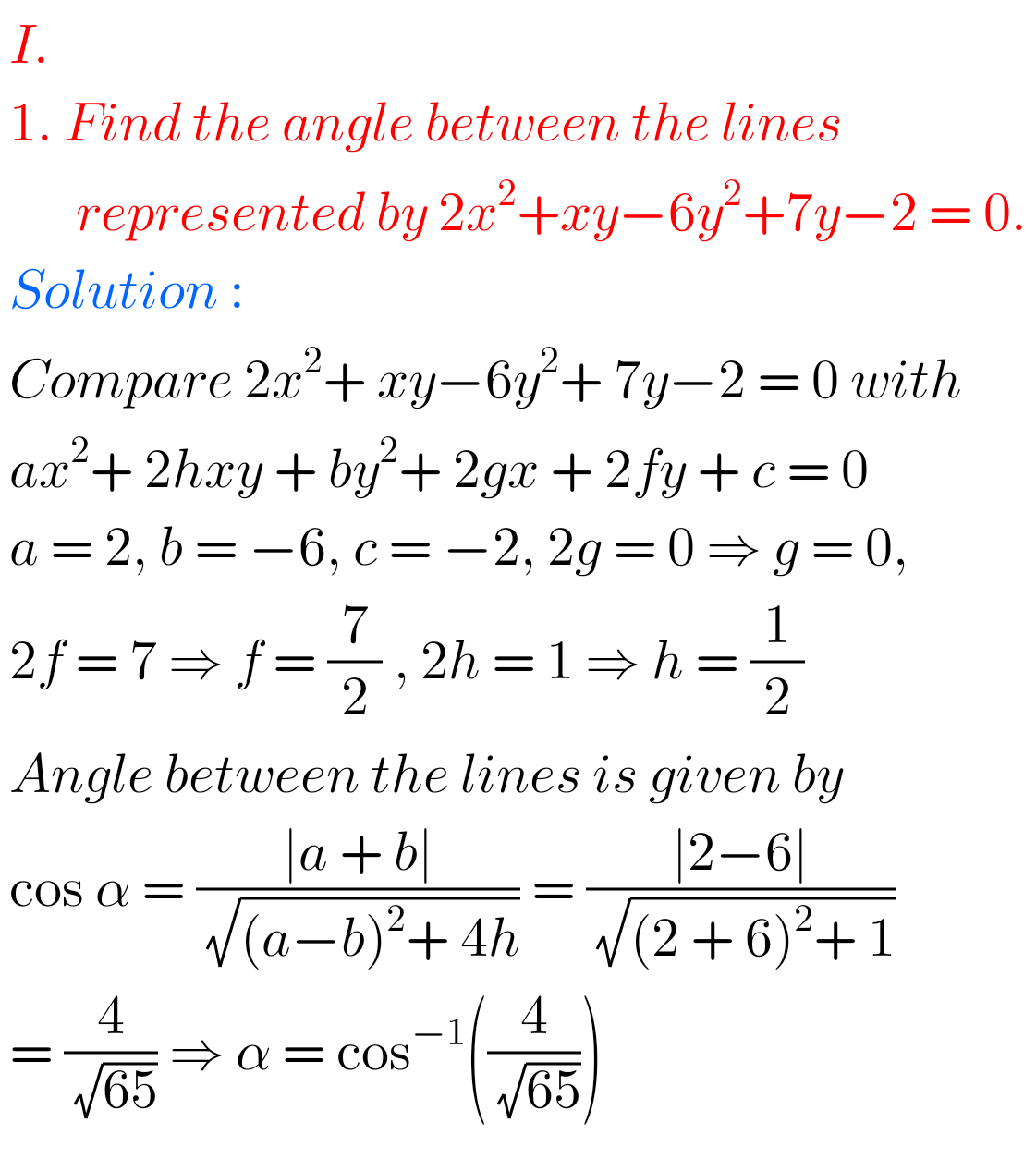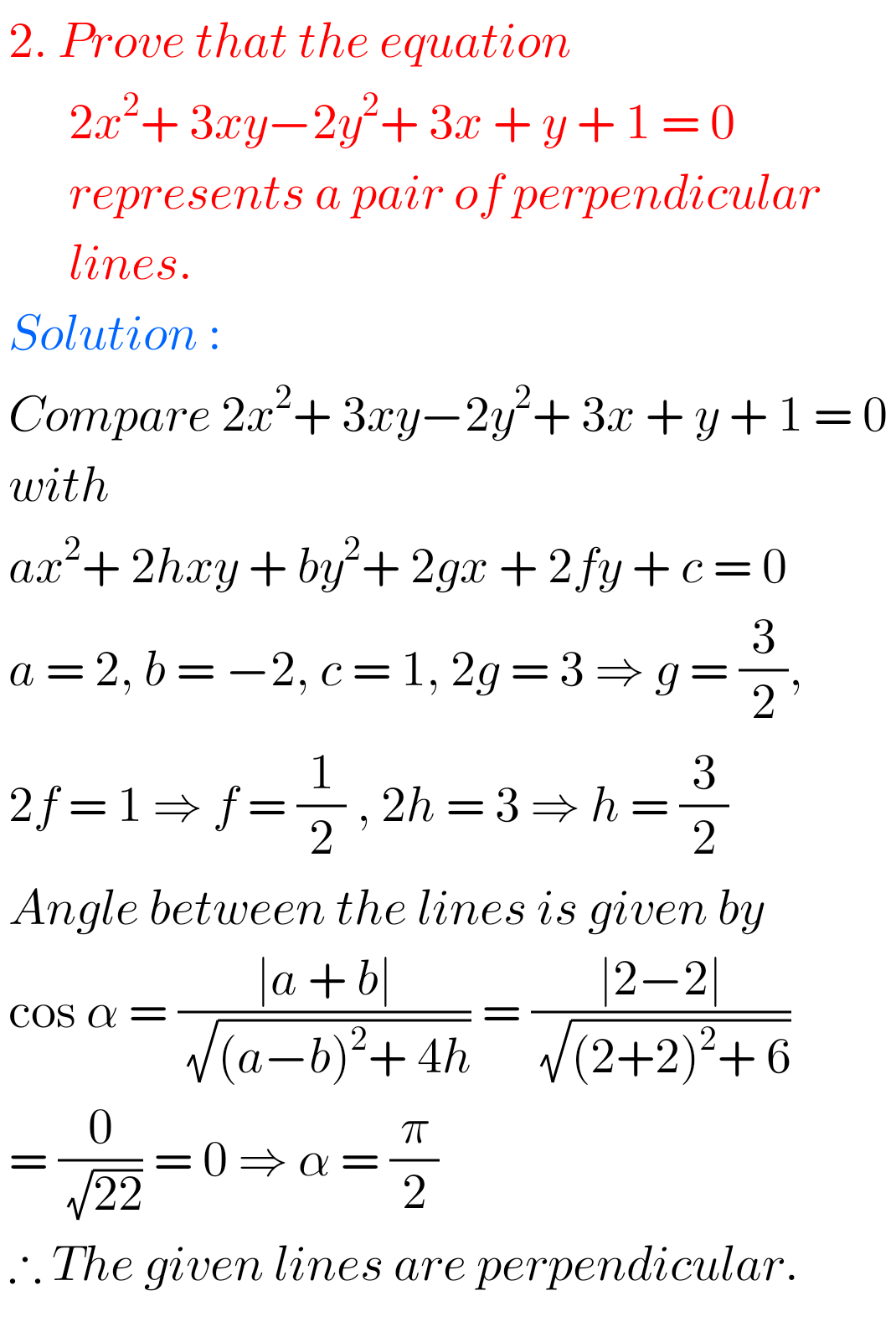## Solutions for Pair of Straight Lines exercise 4(b)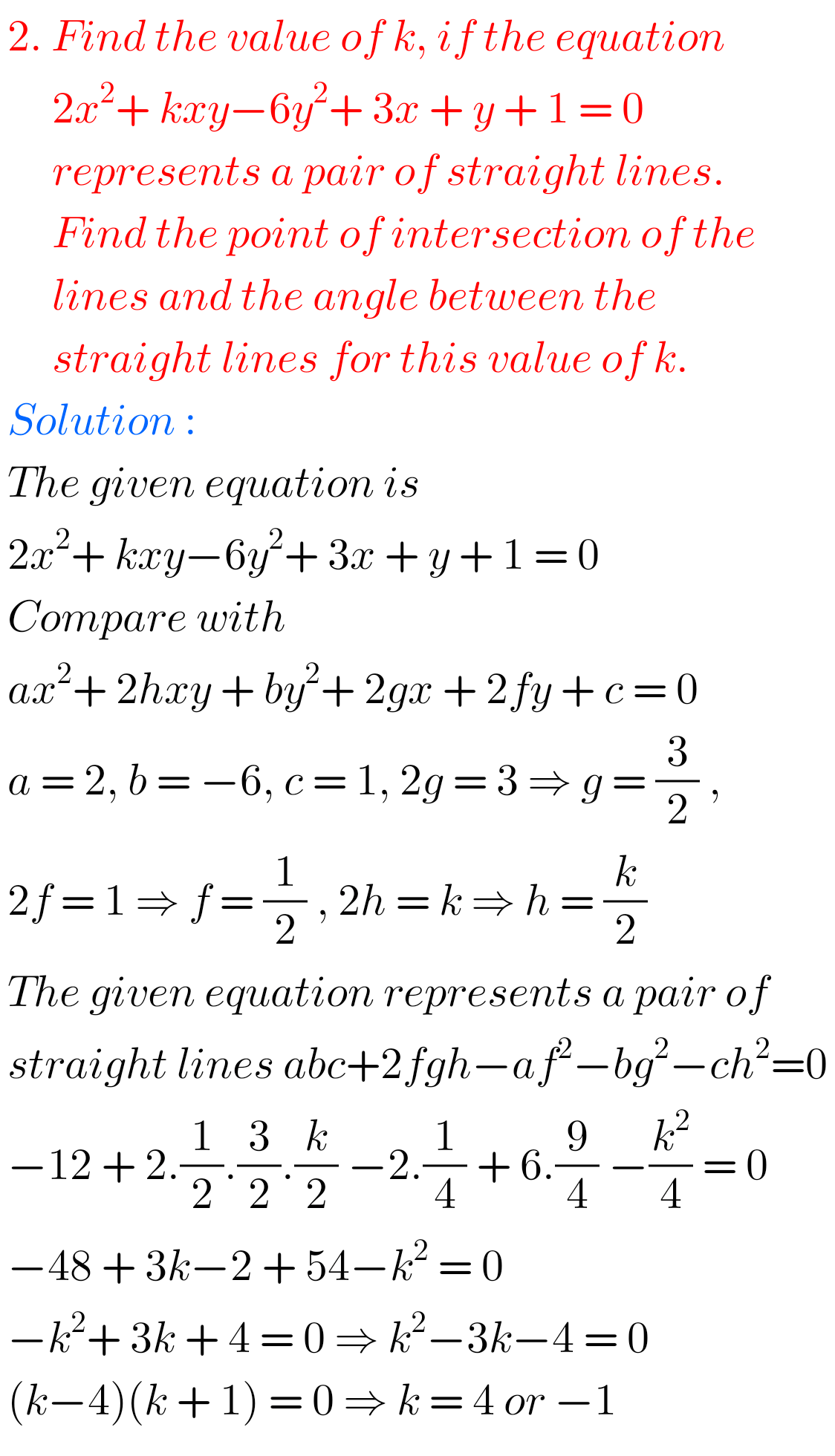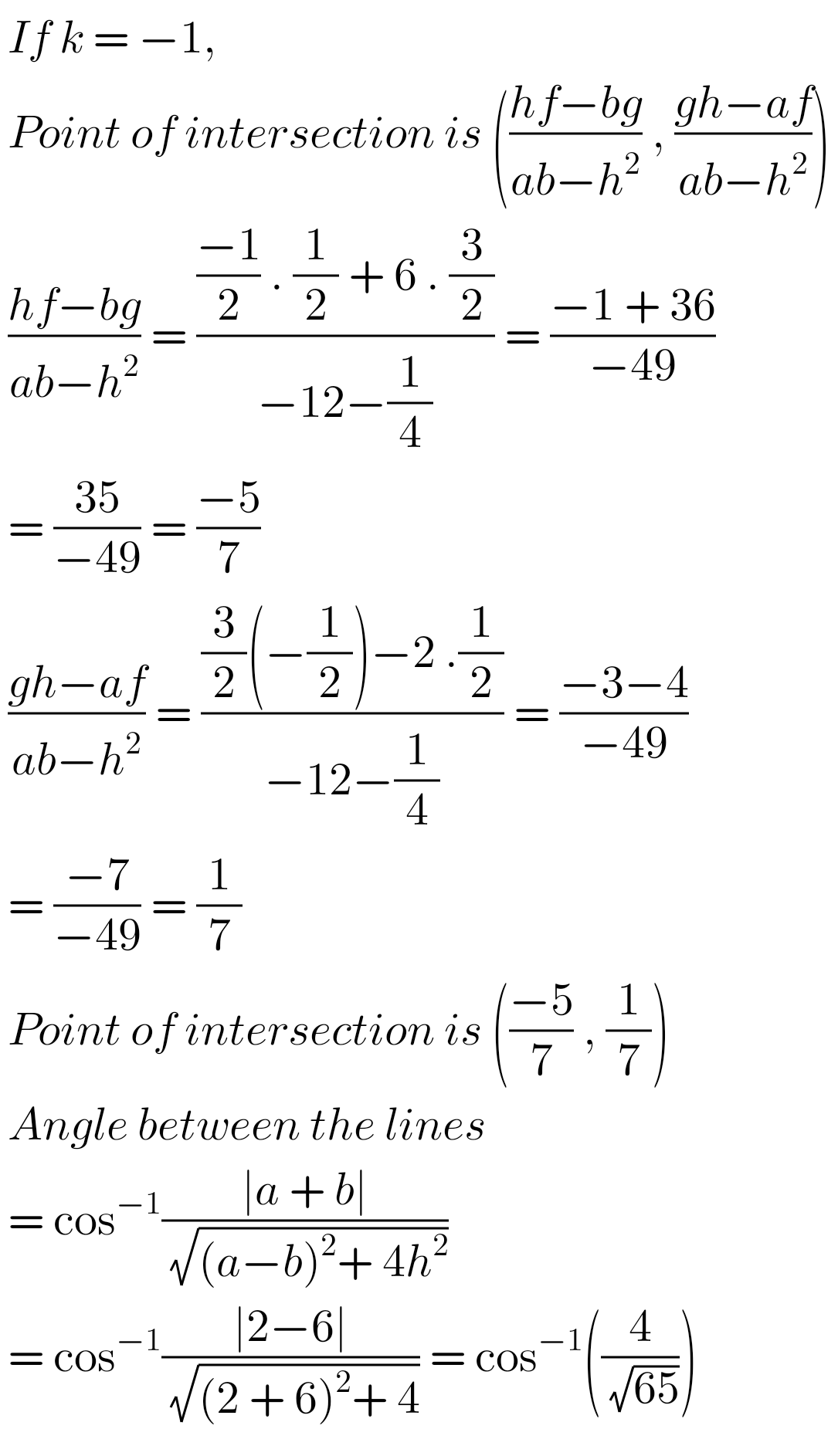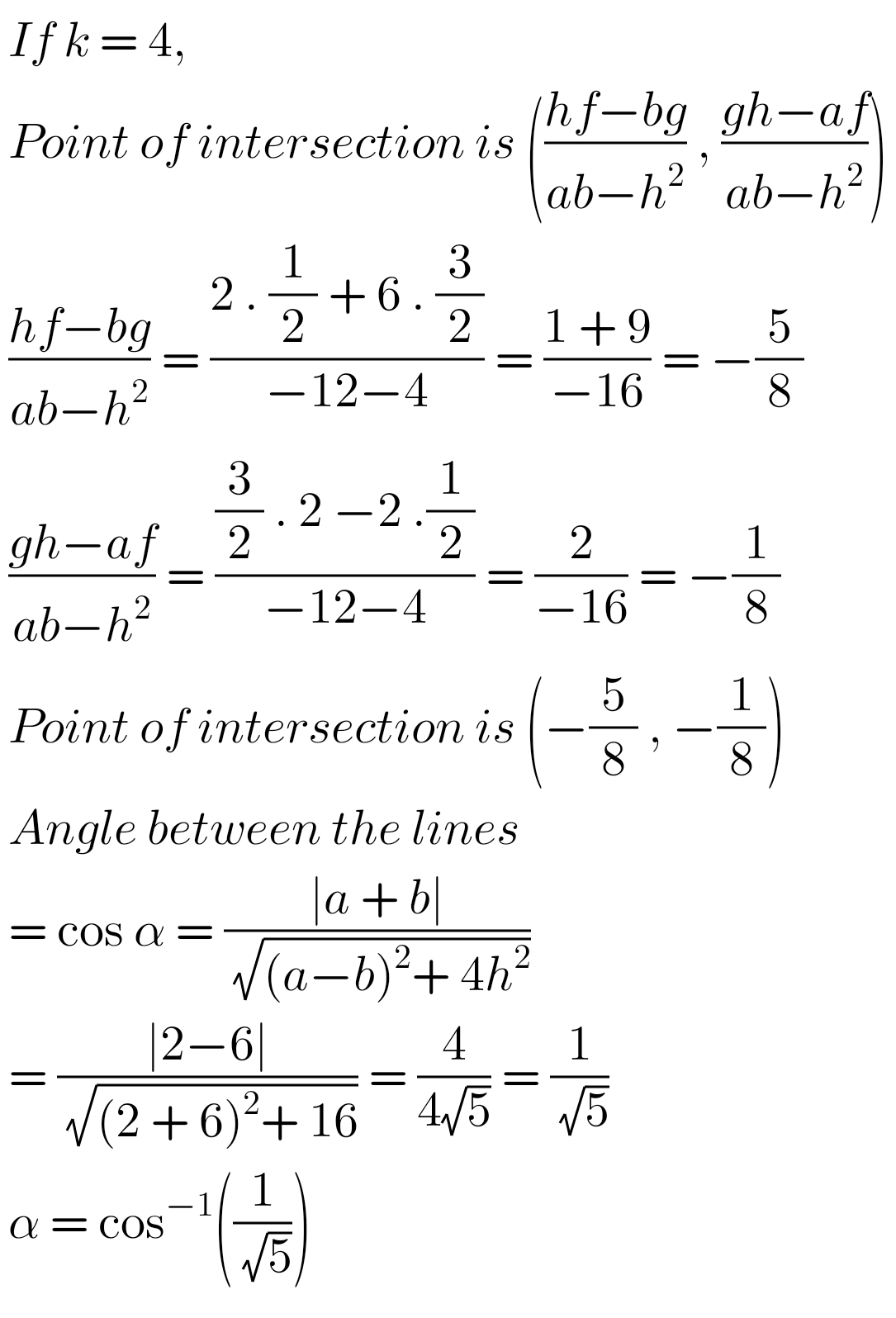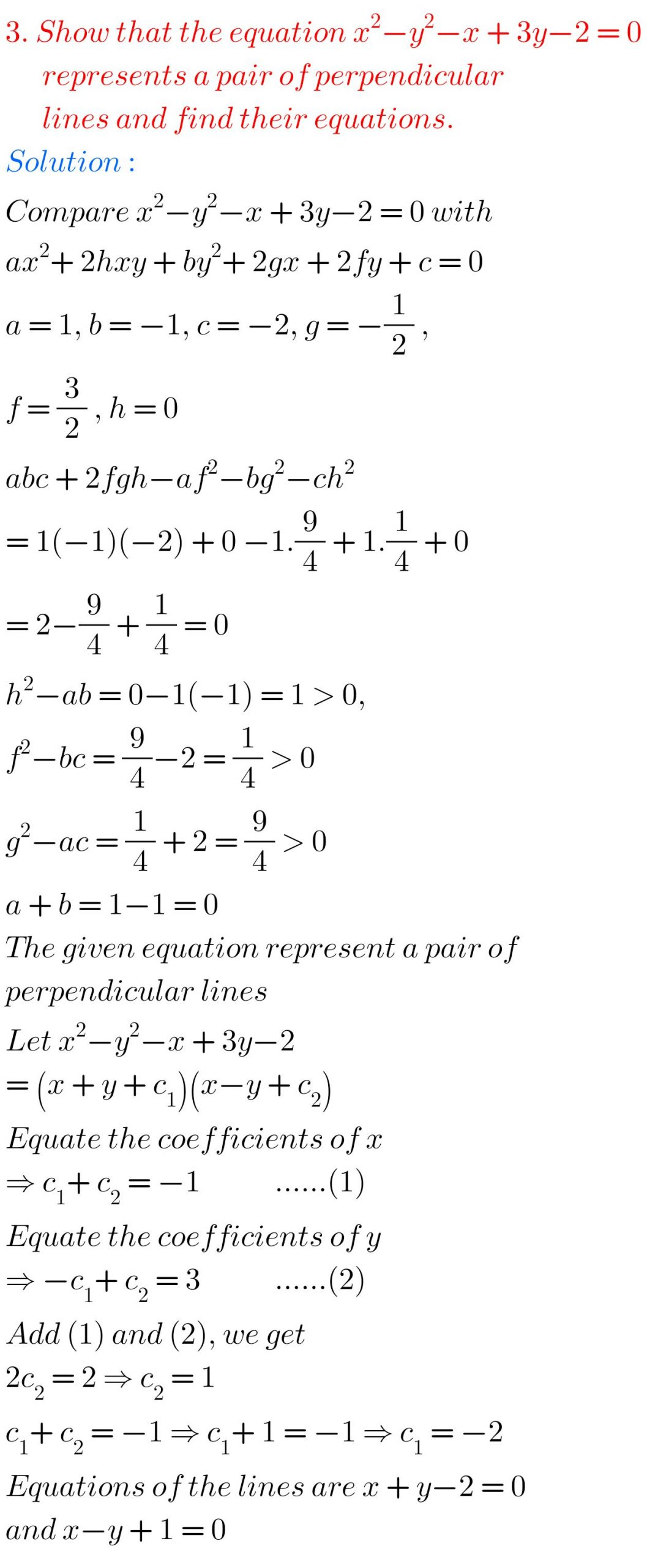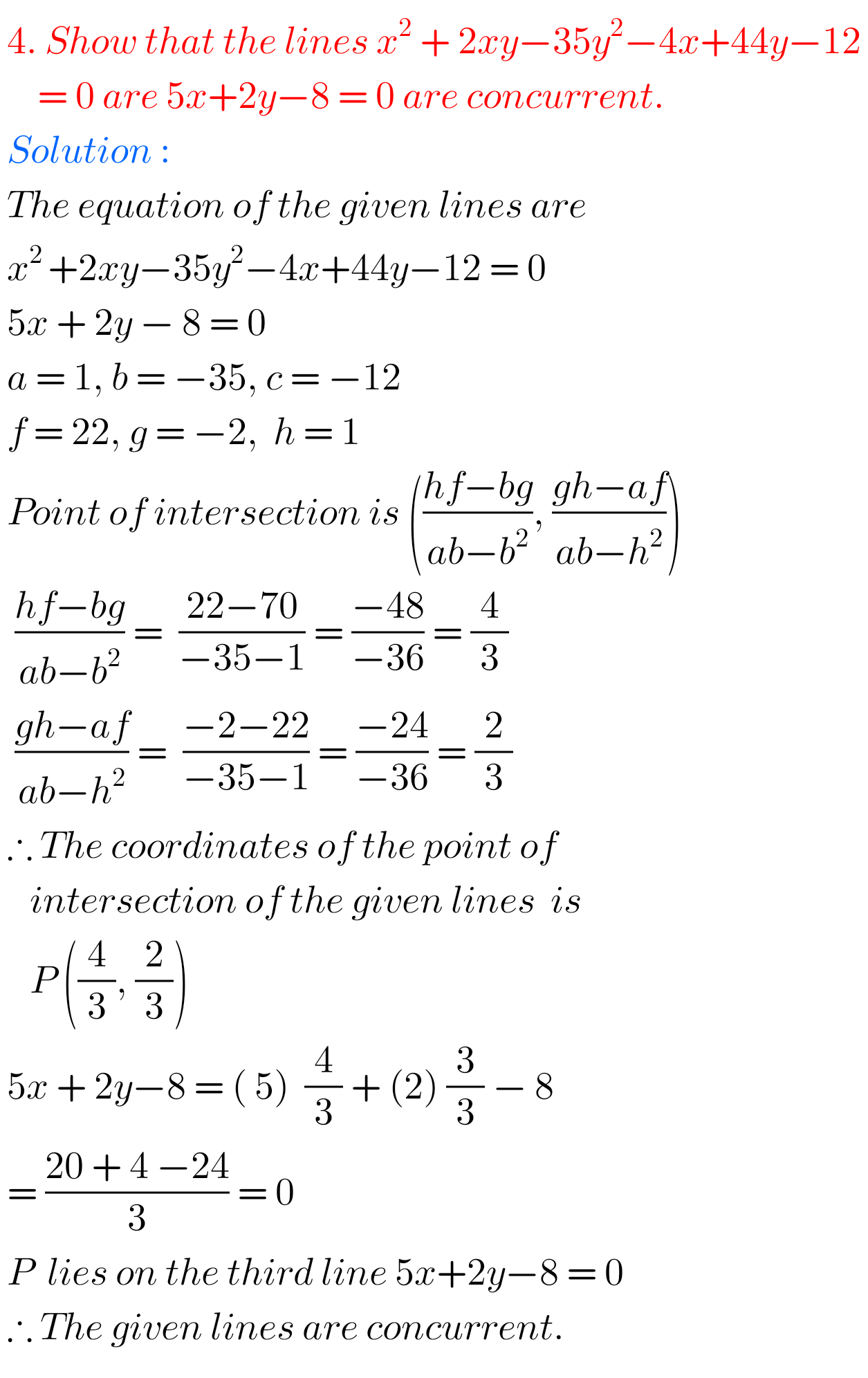F

##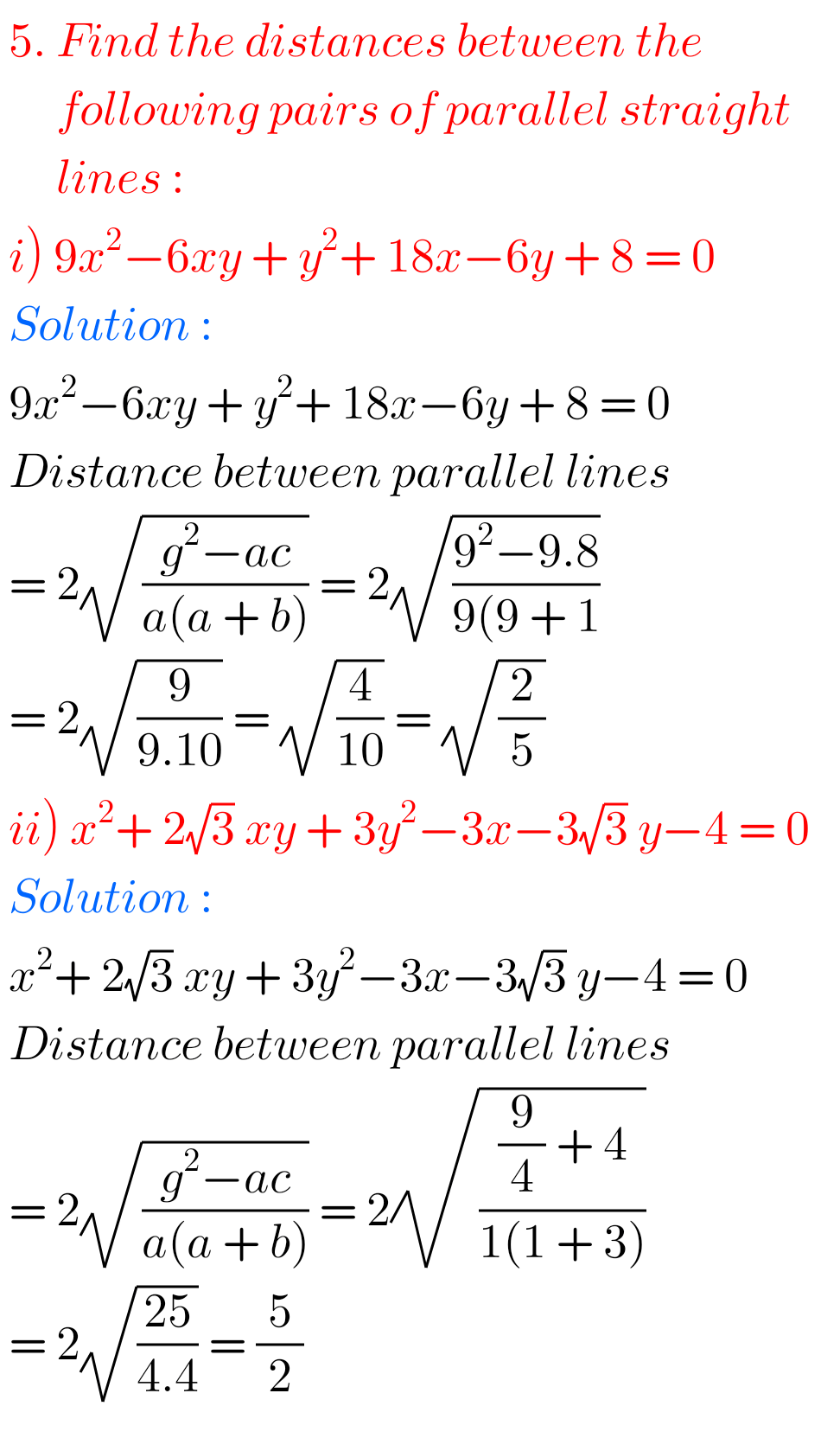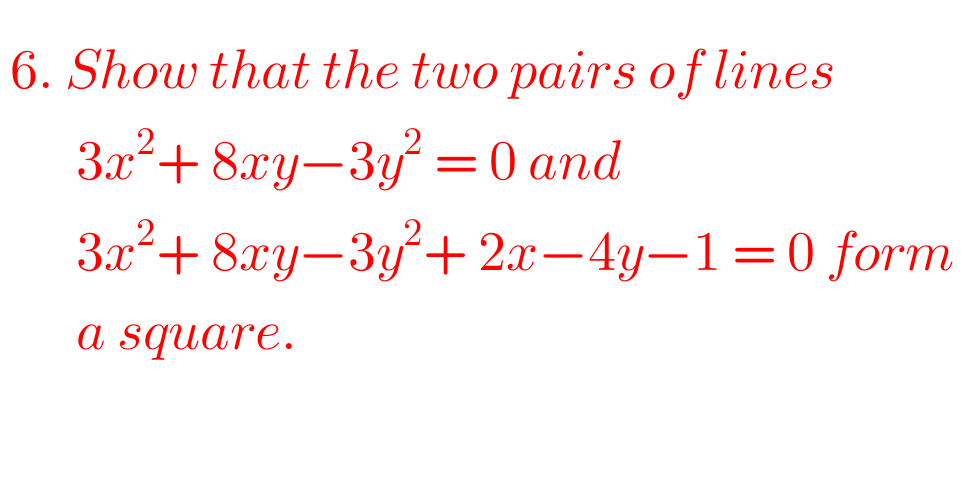F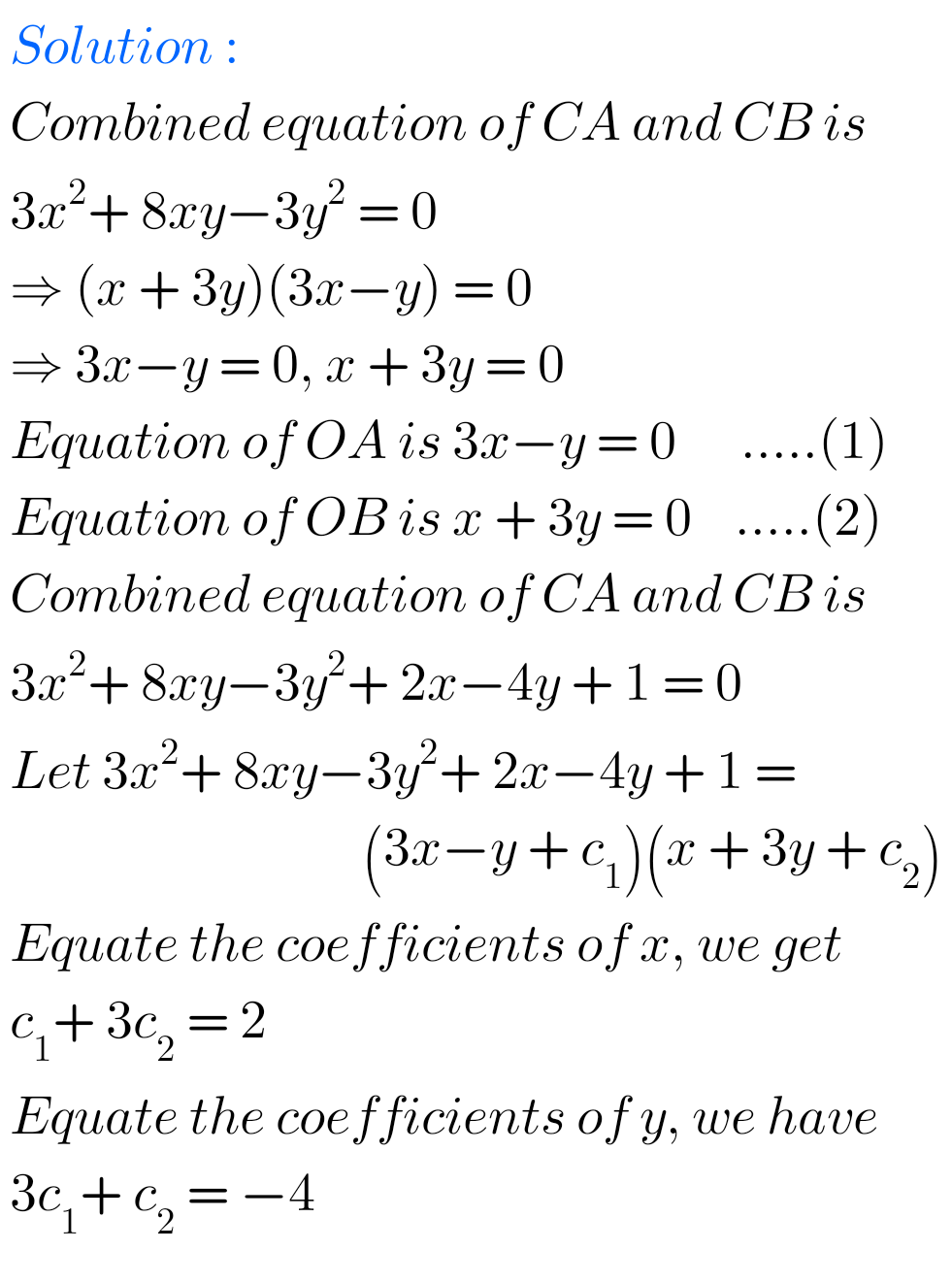F## AP & TS Inter Maths 1B Solutions for Pair of Straight Lines exercise 4(b)F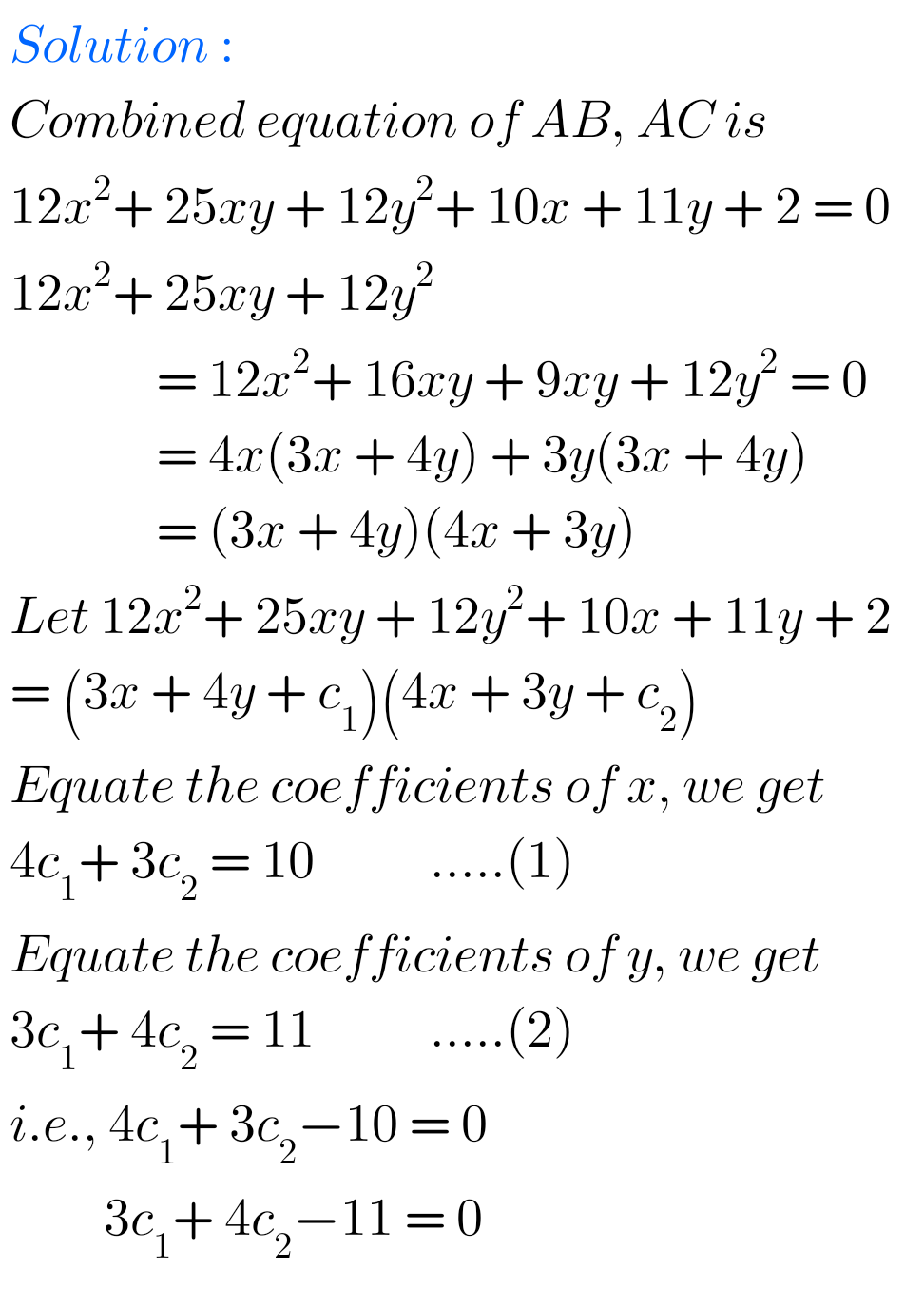FF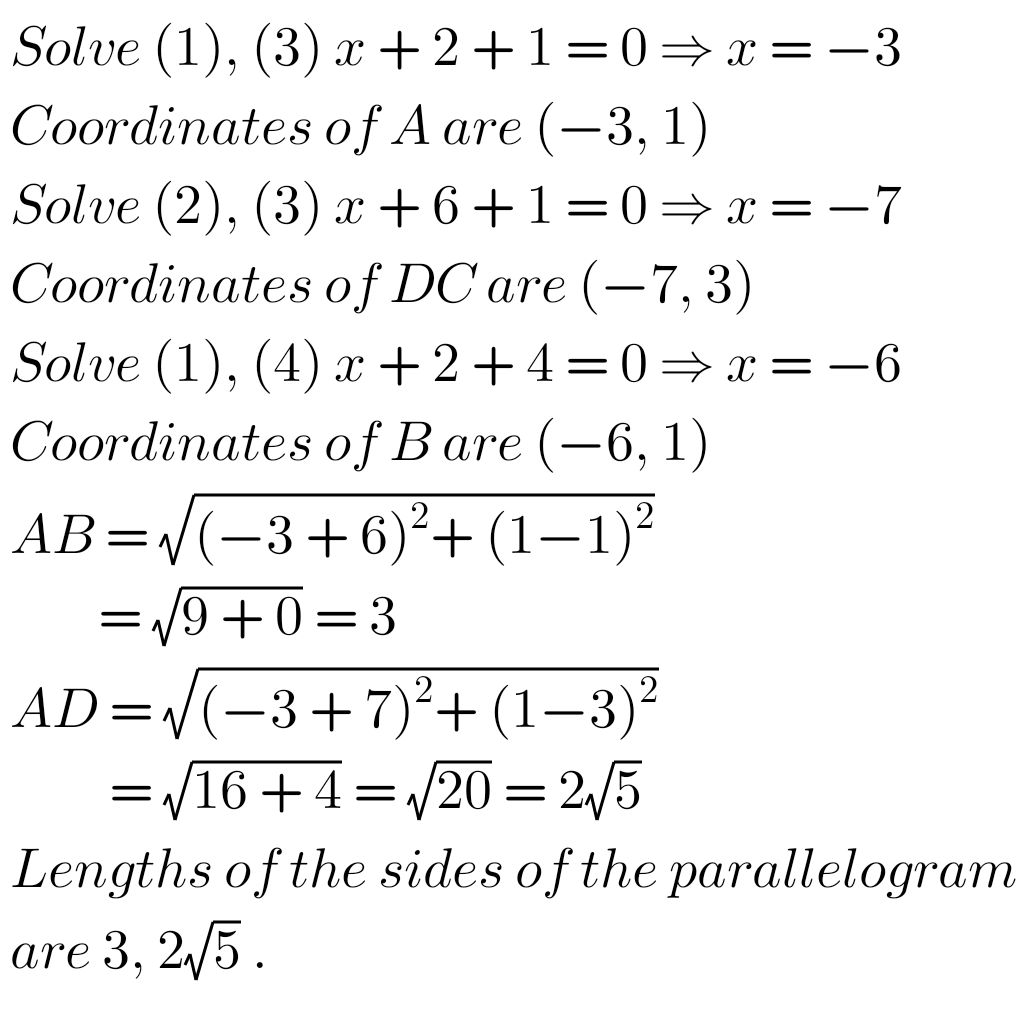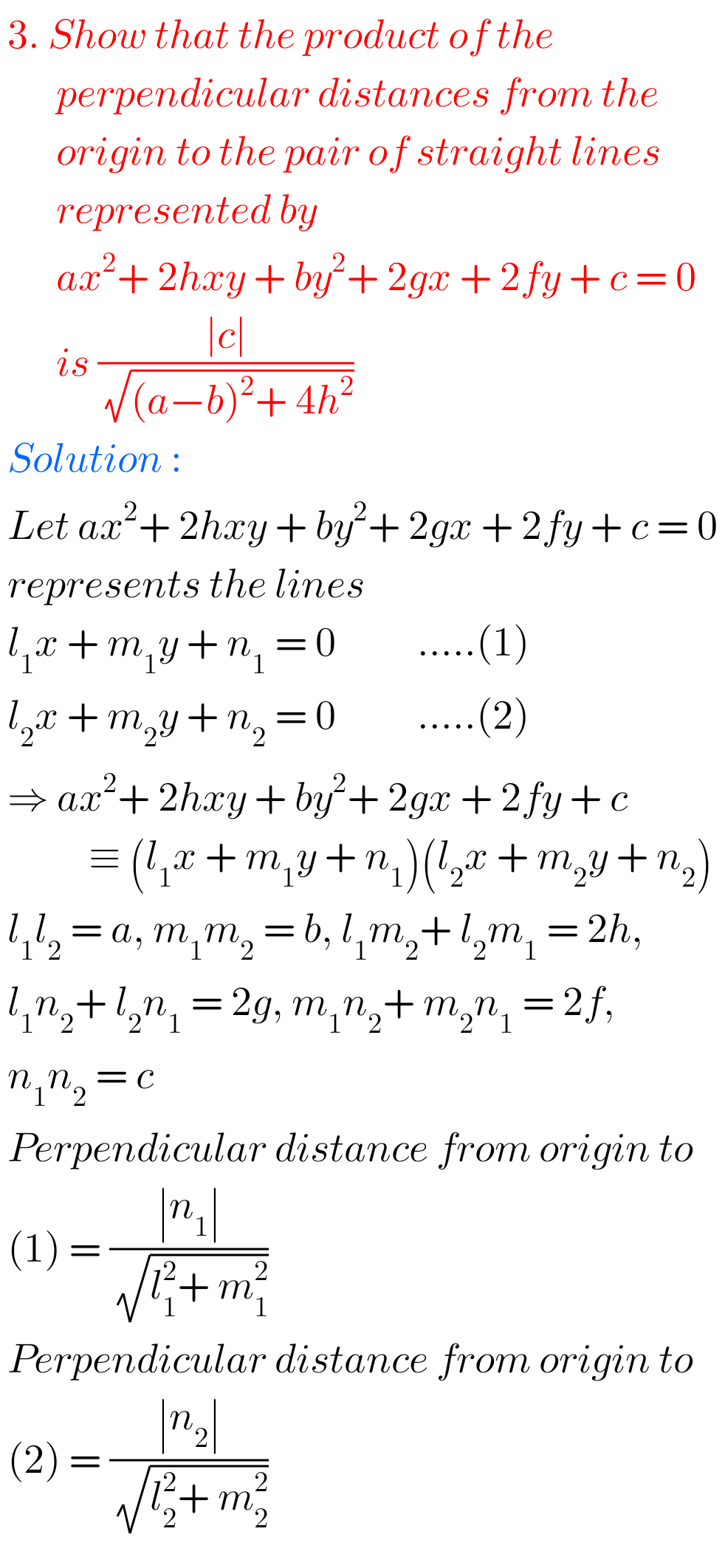Figure for

II. 4.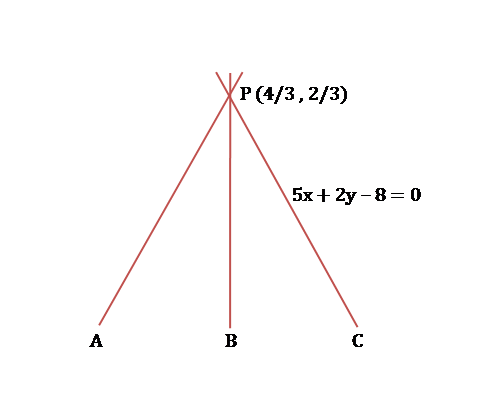Figure for

II. 6.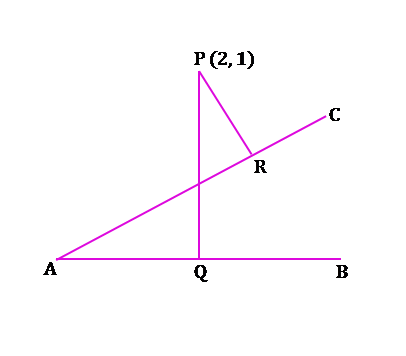Figure for

III. 1.Figure for

III . 2.Note : Observe the solutions and try them in your own method.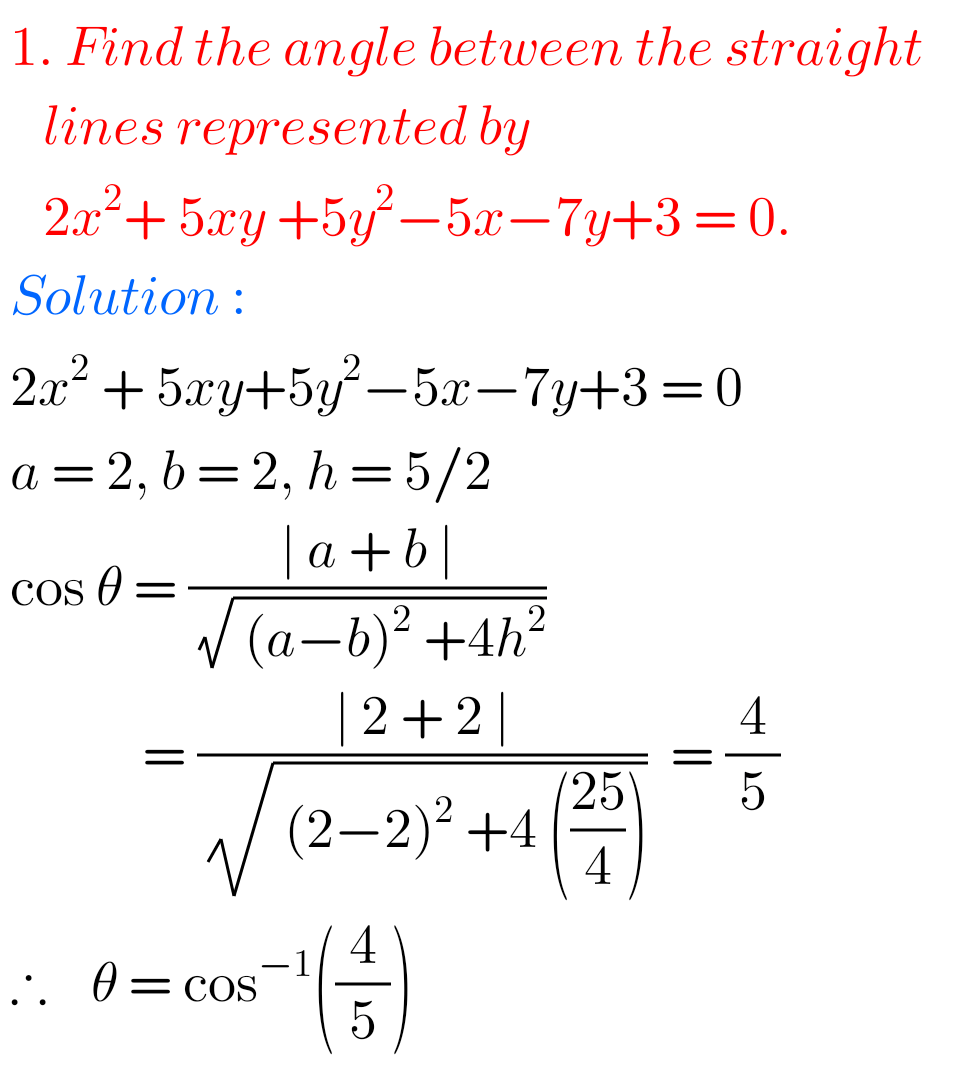Inter 1a exercise 6(a) solutions

class 10 maths polynomials solutions

Ncert maths class 8 exponents and powers

Ncert maths class 7 fractions and decimals

Ncert maths class 6 playing with numbers